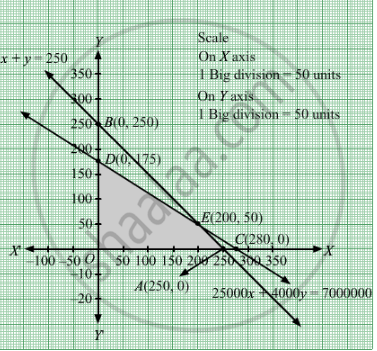# A Merchant Plans to Sell Two Types of Personal Computers a Desktop Model and a Portable Model that Will Cost Rs 25,000 and Rs 40,000 Respectively. - Mathematics

Sum

A merchant plans to sell two types of personal computers a desktop model and a portable model that will cost Rs 25,000 and Rs 40,000 respectively. He estimates that the total monthly demand of computers will not exceed 250 units. Determine the number of units of each type of computers which the merchant should stock to get maximum profit if he does not want to invest more than Rs 70 lakhs and his profit on the desktop model is Rs 4500 and on the portable model is Rs 5000. Make an LPP and solve it graphically.

#### Solution

Let x and be the number of desktop model and portable model respectively.
Number of desktop model and portable model cannot be negative.

Therefore, x ≥ 0 , y ≥ 0 ,

It is given that the monthly demand will not exist 250 units.

∴ $x + y \leq 250$

Cost of desktop and portable model is Rs 25,000 and Rs 40,000 respectively.

Therefore, cost of x desktop model and y portable model  is Rs 25,000 and Rs 40,000 respectively and he does not want to invest more than Rs 70 lakhs.

$25000x + 40000y \leq 7000000$

Profit on the desktop model is Rs 4500 and on the portable model is Rs 5000. Therefore, profit made by x desktop model and y portable model is Rs 4500x and Rs 5000y respectively.

Total profit = Z = 4500x + 5000y

The mathematical form of the given LPP is:

Maximize Z = 4500x + 5000y

Subject to constraints: $x + y \leq 250$

$25000x + 40000y \leq 7000000$
x ≥ 0 , y ≥ 0 ,
First we will convert inequations into equations as follows:
x + y = 250, 25000x + 40000y = 7000000, x = 0 and y = 0

Region represented by x + y ≤ 250:
The line x + y = 250 meets the coordinate axes at A(250, 0) and  $B\left( 0, 250 \right)$  respectively. By joining these points we obtain the line  x + y = 250. Clearly (0,0) satisfies the x + y = 250. So, the region which contains the origin represents the solution set of the inequation x + y ≤ 250.

Region represented by 25000x + 40000y ≤ 7000000:
The line 25000x + 40000y = 7000000 meets the coordinate axes at C(280, 0) and  $D\left( 0, 175 \right)$  respectively. By joining these points we obtain the line 25000x + 40000y = 7000000.  Clearly (0,0) satisfies the inequation 25000x + 40000y ≤ 7000000. So,the region which contains the origin represents the solution set of the inequation 25000x + 40000y ≤ 7000000.

Region represented by ≥ 0 and y ≥ 0:
Since, every point in the first quadrant satisfies these inequations. So, the first quadrant is the region represented by the inequations x ≥ 0, and y ≥ 0.
The feasible region determined by the system of constraints x + y ≤ 250, 25000x + 40000y ≤ 7000000, x ≥ 0 and y ≥ 0 are as follows.The corner points are O(0, 0), D(0, 175), E(200, 50) and A(250, 0).

The values of the objective function Z at corner points of the feasible region are given in the following table:

 Corner Points Z = 4500x + 5000y O(0, 0) 0 D(0, 175) 875000 E(200, 50) 1150000 ← Maximum A(250, 0) 1125000

Clearly, Z is maximum at x = 200 and = 50 and the maximum value of Z at this point is 1150000.

Thus, 200 desktop models and 50 portable units should be sold to maximize the profit.

Concept: Graphical Method of Solving Linear Programming Problems
Is there an error in this question or solution?

#### APPEARS IN

RD Sharma Class 12 Maths
Chapter 30 Linear programming
Exercise 30.4 | Q 41 | Page 55